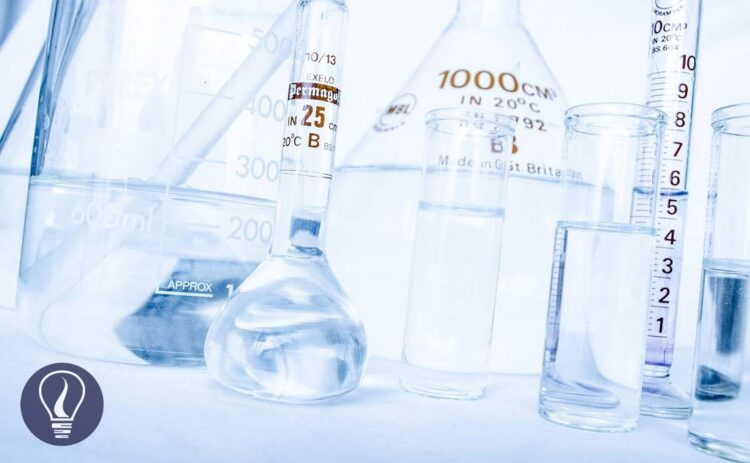More results...

Generic selectors
Exact matches only
Search in title
Search in content
Post Type Selectors

# Ion ActivityB.Sc (Hons, USJ) (Polymer Science and Technology, Chemistry, Physics)
Last Updated On : January 20, 2023
Published Date : December 13, 2022

## Introduction to Ion Activity

When ionic compounds are dissolved in water, they will separate into cations (positively charged ions) and anions (negatively charged ions). In an aqueous media, these ions will be surrounded by water molecules. In an H2O molecule, there is a slight positive charge on the Hydrogen atom and a slight negative charge on the Oxygen atom. Therefore, water molecules will bind to cations through oxygen atoms and anions through hydrogen atoms. Each cation and anion is surrounded by a specific number of water molecules. As an example,

• The 1st coordination sphere of the Li cation (this is a small cation) contains four H2O molecules at the corners of a tetrahedron.
• The 1st coordination sphere of the Cf3+ (this is a large cation) ion appears to be a square antiprism with ~ eight H2O molecules.
• Cl binds with ~ six water molecules (through hydrogen atoms). Water molecules exchange between bulk solvent and ion coordination sites rapidly.

water molecules will bind more tightly to small and more highly charged ions.

## Activity

The activity of an ion is a measure of its effective concentration in a real solution. It gives a proper idea of the chemical potential of each species in a real solution. In an ideal solution, ions remain in their original state. (Not as hydrated species). Therefore, the equilibrium constant can be written as the below expression.

But in a real situation, ions will be hydrated. After water molecules bind to ions, the radius of ions will change. More water molecules will bind to small and highly charged ions. The hydrated ion has a larger radius than the original ion and it will behave as larger species in the solution. Since those ions behave as hydrated species, their effective concentration and chemical potential are changed over their original ion. Therefore, the real activity of a species in a solution can be written as follows.

Where,

• A = Activity of the component
• γ = activity coefficient
• [C] = concentration of the component

In a real solution equilibrium constant will be written as follows.

## Activity coefficient

Activity coefficient gives a measure of the deviation behavior of a solution from its ideal solution. The maximum value for the activity coefficient is. If the activity coefficient is "1" means that the solution behaves as ideal.

A theory has been developed in 1923 by Peter Debye and Erich Hückel to calculate the mean ionic activity coefficient in a solution. Debye Huckel's theory is based on three assumptions.

• In a solution, electrolytes will dissociate into ions completely.
• Solutions of Electrolytes are considered very dilute solutions, on the order of 0.01 M.
• On average, each ion in the solution is surrounded by ions with opposite charges.

According to the above theory, the ionic activity coefficient is expressed as,

Where,

• γ = ionic activity coefficient
• z = ionic charge of the electrolyte
• μ = ionic strength
• α = size of the ion in picometers

## Ionic strength

When a saturated ionic solution is added to an inert salt, (ions in salt will not react with the ions in the solution) the solubility of the ionic substance will be increased. Let’s consider a saturated solution of CaSO4.

At 25℃ dissolved species of the above system are Ca2+ (0.01M), SO42- (0.01M), and CaSO4 (0.005M) as ion pairs. When the above system is added KNO3 (salt), the system is given K+ ions and NO3- ions. But neither K+ nor NO3- will react with either Ca2+ or SO42- ions. But after adding the salt, more solid CaSO4 will dissolve in water and the concentrations of Ca2+ and SO42- ions will be increased by 30%.

This phenomenon is called the “salt effect”. The salt effect can be explained by considering the ionic strength changes of the solution.

Ionic strength is a measure of the concentration of ions in an electrolyte solution. Ionic strength is

Where,

• μ = ionic strength
• z = ionic charge of the electrolyte
• C = concentration of each ion

## Ion pairs

When a salt dissolves in water it will dissociate into cations and anions. In less than 0.1M concentrations, salts that are composed of ±1 charged ions will almost completely dissolve in water. But salts that contain ±2 charged ions will dissociate less even in a dilute solution. Such salts form ion pairs. Ion pair is a temporary attraction between positive and negatively charged ions.## References and Attributes

### Figures:

The cover image was created using an image by PublicDomainPictures from Pixabay